Command Reference : Operator and Function Reference : Time Series Functions

Time Series Functions
The following functions facilitate working with time series data. Note that NAs will be returned for observations for which lagged values are not available. For example, d(x) returns a missing value for the first observation in the workfile, since the lagged value is not available.

 Name Function Description @bridge(s) imputes NAs in s. Returns a copy of series s with NAs replaced by the nearest preceding non-NA value. This function is panel aware. d(x) first difference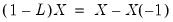where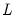is the lag operator. d(x,n) n-th order difference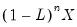. d(x,n,s) n-th order difference with a seasonal difference at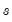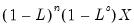. dlog(x) first difference of the logarithm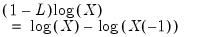. dlog(x,n) n-th order difference of the logarithm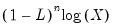. dlog(x,n,s) n-th order difference of the logarithm with a seasonal difference at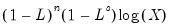. @demean(s[, smpl]) demean Returns a copy of series s translated to have a mean of zero. @demeanby(s, g[, smpl]) demean by group Returns a copy of series s translated to have a mean of zero within each group of observations defined by series g. @detrend(s[, smpl]) detrend Returns the residuals of an OLS regression on series s versus an implicit time trend. This function is panel aware. @fracdiff(s, n) returns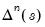for series s (see description). This function is panel aware. Fractional difference operator: For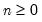,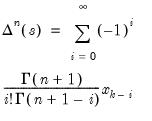@lag(x, n) n-th order lag (equivalent to “X(‑4”) for the series X)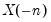@pc(x) one-period percentage change (in percent) equals @pch(x)*100 @pch(x) one-period percentage change (in decimal)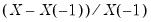. @pca(x) one-period percentage change—annualized (in percent) equals @pcha(x)*100 @pcha(x) one-period percentage change—annualized (in decimal)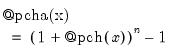where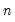is the lag associated with one-year (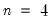) for quarterly data, etc.). @pcy(x) one-year percentage change (in percent) equals @pchy(x)*100 @pchy(x) one-year percentage change (in decimal)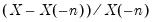, whereis the lag associated with one-year (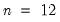) for annual data, etc.). @periodtodate(s, p[, smpl]) period-to-date of series s. Cumulative sum of series s within each period, where each period is defined by a contiguous block of identical values in series p. @ytd(s[, smpl]) YTD of series s. Provides the cumulative sum of a series within the year. Optionally, a sample may be provided to limit the observations included in the summation.